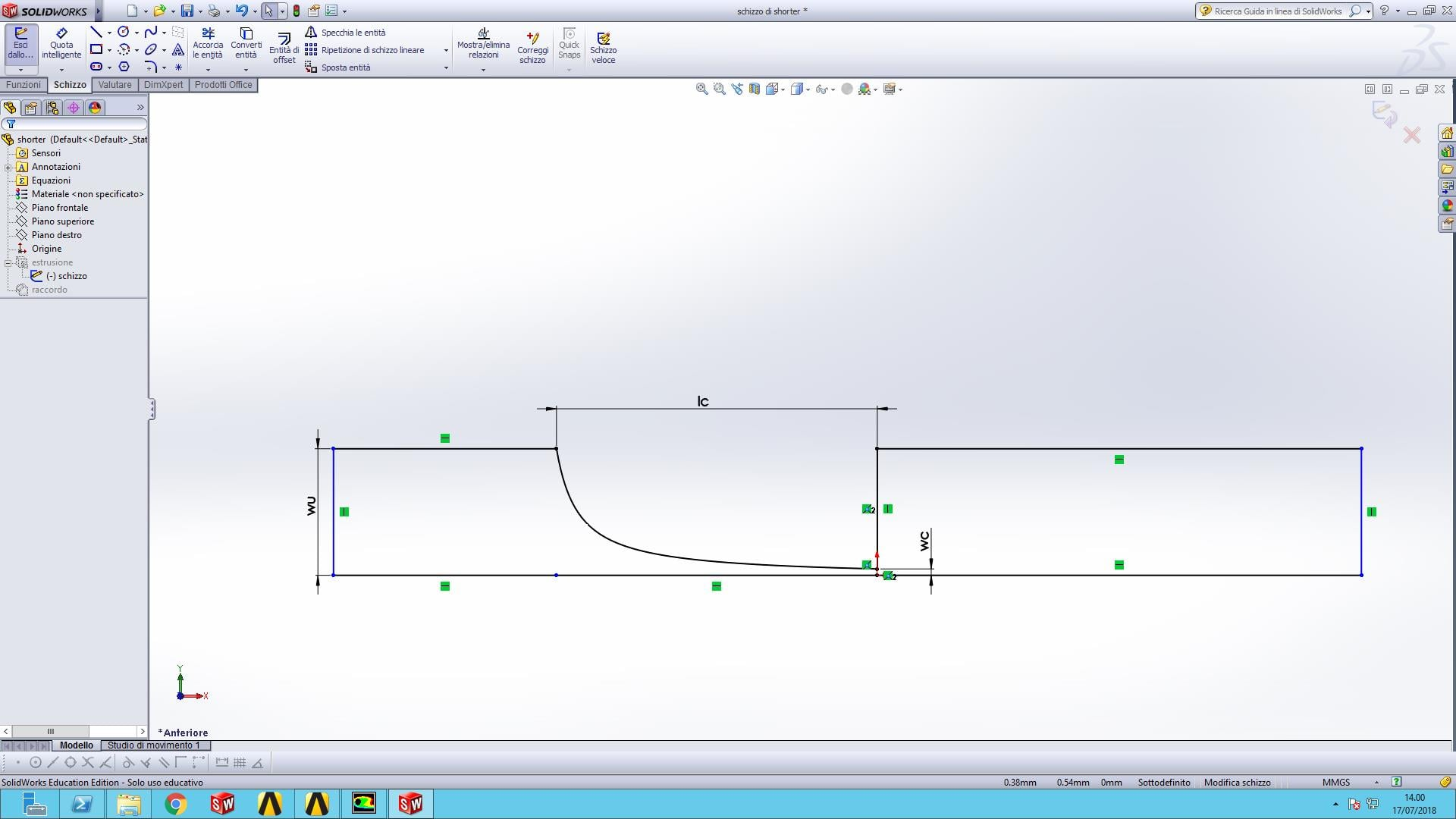Valeria
Subscriber

yes I can but WB seems to be unable to update the parameters of the hyperbola probably because its equation is set in solidworks. This is a screen of what I'd like to draw inside design modeller, but I can't figure out how to set the cone angle and psition of the plane to intersect it in orded to obtain precisely the hyperbola of equation

y=0.2/(1+29.32(x+0.65))